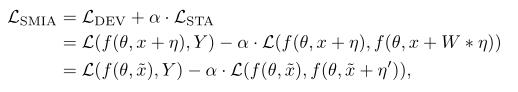# Multiple losses, ignored when multiplied by scalar

Hi,
I try to implement a stabilized attack method, which consist of a classical iterative fast gradient sign method (FGSM) plus a stabilization term.

Here is the definition of the loss stabilized (W is gaussian kernel, eta the sign grad through iteration) :Below my implementation

``````def smia_attack(image, epsilon, alpha, model, target, n_step):

L_dev = F.nll_loss(model(attack_image), target)
L_dev.backward()

W = gaussianBlur(10, 3, 1).cuda()
for i in range(n_step):
L_smia = F.nll_loss(model(attack_image), target) - alpha * F.nll_loss(model(attack_image), model(attack_image + W(eta) - eta).argmax().unsqueeze(0))
L_smia.backward()
return attack_image + eta
``````

When I generate perturbed images, Pytorch seems to ignore the right parrt of L_smia, namely : -alpha *F.nll_loss (…) because if I remove it, nothing changes, and I have the exact same result without, ie, it’s doing vanilla FGSM.
However, if I remove the alpha term, then the result is pretty different, Pytorch seems to make us of it.

Any idea why ?

Hi,

Note that it would be better to replace:

``````        L_smia.backward()
``````        grad, = autograd.grad(L_smia, attack_image)
Also you can check the gradients flowing back by using hooks: on any Tensor, you can set `t.register_hook(your_fn)`. So that your_fn will be called for the gradient for that Tensor. That way you can check what are the gradient values flowing on the right part of your model.